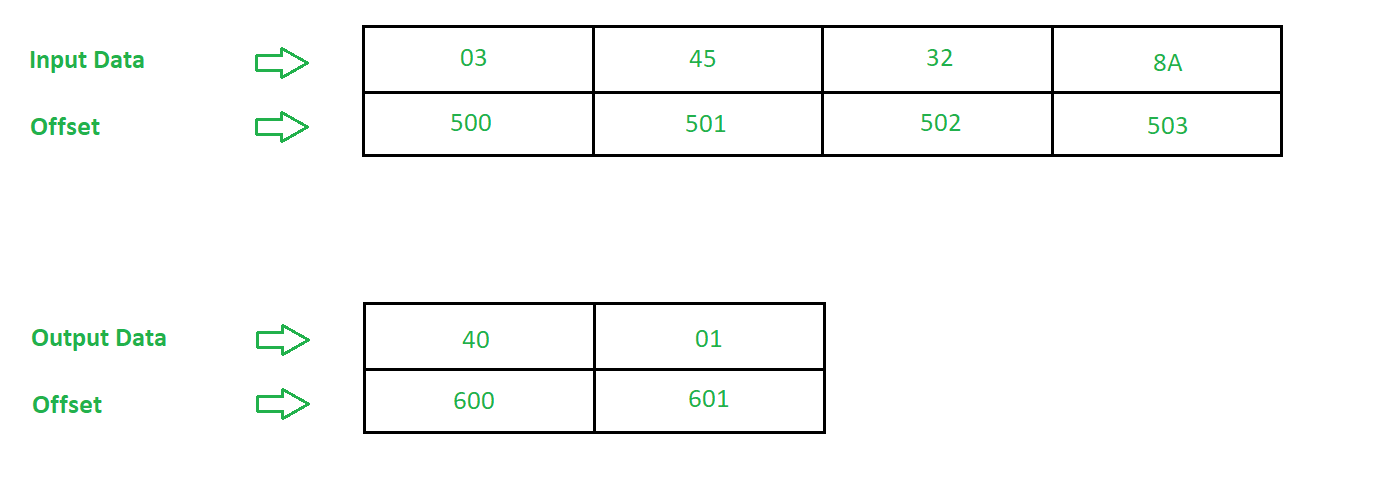# 8086 program to find average of n numbers

• Difficulty Level : Medium
• Last Updated : 17 May, 2018

Problem – Write an assembly language program in 8086 microprocessor to find average of n eight bit numbers.

Example –Algorithm –

1. Assign value 500 in SI and 600 in DI
2. Move the contents of [SI] in CL
3. Move 0000 in AX
4. Move the contents of CL to BL
5. Increment the value of SI by 1
6. Add the contents of AL and [SI]
7. Add 00 to AH with previous carry
8. Increment the value of SI by 1
9. Decrements the value of CL by 1
10. If Zero Flag (ZF) is not set go to step 6 else go to step 11
11. Divide the contents of AX by BL
12. Move the contents of AX in [DI]
13. Halt the program

Program –

OFFSETMNEMONICSCOMMENT
400MOV SI, 500SI <- 500
403MOV DI, 600DI <- 600
406MOV AX, 0000AX = 0000
409MOV CL, [SI]CL <- [SI]
40BMOV BL, CLBL <- CL
40DINC SISI = SI + 1
40EADD AL, [SI]AL = AL + [SI]
410ADC AH, 00AH = AH + 00 + cy
412INC SISI = SI + 1
413DEC CLCL = CL – 1
415JNZ 40EJUMP if ZF = 0
417DIV BLAX = AX / BL
419MOV [DI], AX[DI] <- AX
41BHLTStop

Explanation –

1. MOV SI, 500 is used to move offset 500 to Starting Index(SI).
2. MOV DI, 600 is used to move offset 600 to Destination Index(DI).
3. MOV AX, 0000 is used to move data 0000 to AX.
4. MOV CL, [SI] is used to move the contents of [SI] to BL.
5. MOV BL, CL is used to copy contents of CL to BL.
6. INC SI is used to increment contents of SI by 1.
7. ADD AL, [SI] is used to add contents of [SI] to AL.
8. ADC AH, 00 is used to 00 along with previous cy to AH.
9. INC SI is used to increment contents of SI by 1.
10. DEC CL is used to decrement contents of CL by 1.
11. JNZ 40E is used to jump to offset 40E if value of ZF = 0.
12. DIV BL is used to multiply contents of AX by BL.
13. MOV [DI], AX is used to move the contents of AX to [DI].
14. HLT stops executing the program and halts any further execution.
My Personal Notes arrow_drop_up